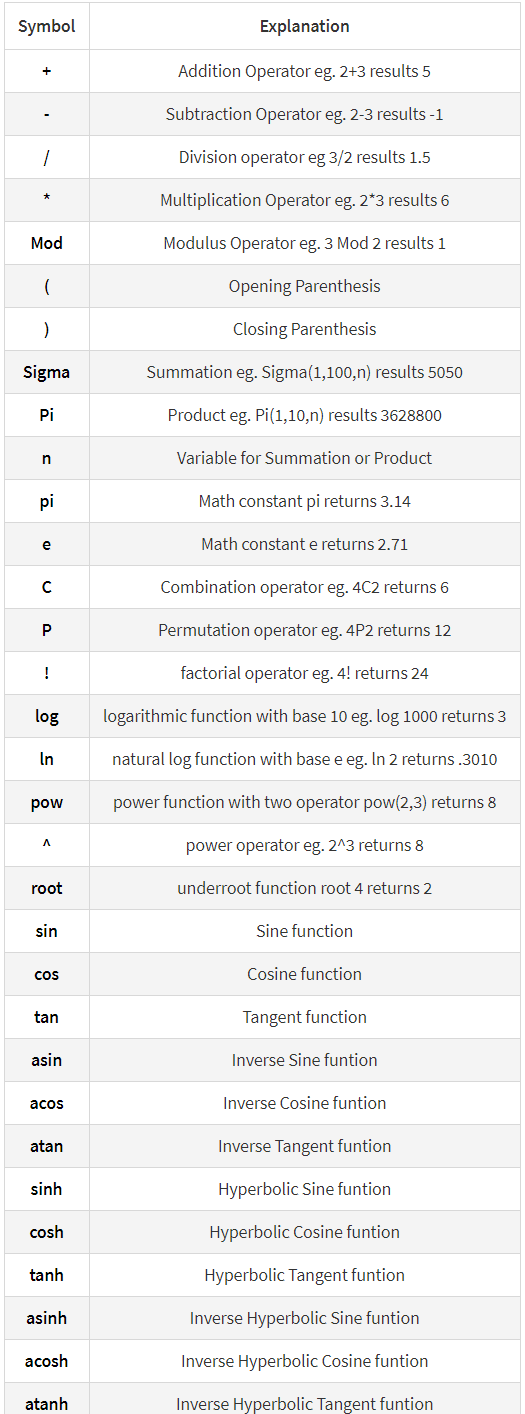# Download WPForms Cost Calculator on Codecanyon

\$25.00

## Description WPForms Cost Calculator :

Download WPForms Cost Calculator. This App releases on Wednesday 12th January 2022 By The author rednumber on Codecanyon. It’s uses with calculations,Cost Calculator,wp forms,wpforms,wpforms Cost Calculator,wpforms number,wpforms number format.

Item Title: WPForms Cost Calculator
Category: wordpress/forms
Price: \$25
Author: rednumber
Published Date: Wednesday 12th January 2022 04:32:50 PM

## WPForms Calculator

Create form calculators, advanced cost estimators extensive for WPForms

With this Add-on for WPForms you can create advanced calculation forms based on the user input. Make awesome booking and order forms or create complex estimation forms. It is as easy as doing normal mathematics.

#### Conditional calculations

if(A < B,A + 1, A +3 ) + 5 + if(number1 < number2, number-600 + 15, 1) +7

#### Dates calculations

days( date2, date1 )
months( date2, date1 )
year( date2, date1 )

### EQUATIONS / FORMULAS FORMAT FOR CALCULATED FIELDS

With simple mathematical operations:

```sin(cos(tan(90))) * Sigma(1,15,n) + 2^3

fieldname1 / fieldname2

(fieldname1 * ( fieldname2 + fieldname3 ) ) / 5

fieldname1 * ( fieldname2 + fieldname3 )
```

## MAIN FEATURES

• Easy use
• Number and Total formats
• Supports conditional calculations
• Support all mathematical formulas
• Creating forms with automatically calculated fields
• Multi Total field
• Cross browser’s compatible
• Custom format total field
• Finance calculators
• Quote calculators
• Booking cost calculators
• And more…

### Supported symbols

```days( date2, date1 )
months( date2, date1)
years( date2,date1 )
floor( number ) : returns the largest integer less than or equal to a given number.
ceil( number) :rounds a number up to the next largest integer.
mod(5,2): returns the division remainder.
min( number1, number 2,....) : returns the lowest-valued number.
max(numbe1, numbe 2, ...): returns the largest number.
```### CHANGELOG

```01/Apr/2022: (version 1.0.0)
- Added: Campatible with "Payments"

13/January /2022: (version 0.1)
- Version 1.0 Initial Release
```

#WPForms #Cost #Calculator

## User Reviews

0.0 out of 5
0
0
0
0
0

There are no reviews yet.